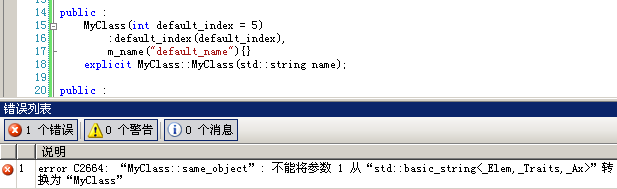# MyMSDN

MyMSDN记录开发新知道

## C++ notes (5)

41、vector、list、deque的性能初窥

```int large_size = 10000000;

cout_current_time("start init vector!\t");
vector<string> svec1(large_size, "Hello");
vector<string> svec2(large_size, "Hi");
cout_current_time("end init vector!\t");

cout_current_time("start init list!\t");
list<string> slist1(large_size, "Hello");
list<string> slist2(large_size, "Hi");
cout_current_time("end init list!\t");

cout_current_time("start init deque!\t");
deque<string> sdeq1(large_size, "Hello");
deque<string> sdeq2(large_size, "Hi");
cout_current_time("end init deque!\t");```

```start init vector!    current time : 5:5:52
end init vector!    current time : 5:5:55
start init list!    current time : 5:5:55
end init list!    current time : 5:6:14
start init deque!    current time : 5:6:14
end init deque!    current time : 5:6:26```

```#include <time.h>
typedef struct tm * time_type;
time_type get_current_time(void) {
time_t t;

t = time(NULL);
return localtime(&t);
}```

42、容器自增长（P286）

43、类定义中为何不能具有自身的数据成员（P375）

Screen window;

};

44、两种引用类类型的方法（P376）

Sales_item item1; //default initialized object of type Sales_item

class Sales_item item1; //equivalent definition of item1

45、为什么类的定义以分号结束（P376）

class Sales_item {/* … */};

class Sales_item {/* … */} accum, trans;

46、形参表和函数体处于类作用域中，函数返回类型不一定在类作用域中

```#include "stdafx.h"
#include <iostream>

class MyClass
{
public :
typedef int index_t;
index_t twice(index_t in);
};

MyClass::index_t MyClass ::twice(index_t in)
{
return in * 2;
}

int _tmain(int argc, _TCHAR* argv[])
{
using namespace std;

MyClass obj;
MyClass::index_t x, y;

x = 10;
y = obj.twice(x);

cout<<"x = "<<x<<"; y = "<<y<<";"<<endl;

return 0;
}```

47、构造函数初始化式（P387）

48、成员初始化的次序

class X{

int i;

int j;

public:

//run-time error: i is initialized before j

X(int val): j(val), i(j) {}

}

49、使用默认构造函数（P393）

Sales_item myobj();

Sales_item myobj(); //ok: but defines a function, not an object

if(myobj.same_isbn(Primer_3rd_ed)) // error: myobj is a function

Sales_item myobj;

Sales_item myobj = Sales_item();

50、显式或隐式初始化

```#include "stdafx.h"
#include <iostream>
using namespace std;
class MyClass
{
public :
typedef int index_t;
bool same_object(MyClass obj);

public :
MyClass(int default_index = 5)
:default_index(default_index),
m_name("default_name"){}
MyClass::MyClass(std::string name);

public :
int default_index;
std::string m_name;
};

MyClass::MyClass(std::string name)
:default_index(0), m_name(name){}

bool MyClass::same_object(MyClass obj)
{
cout<<"m_name = "<<m_name.c_str()<<endl;
cout<<"obj.m_name = "<<obj.m_name.c_str()<<endl;
return strcmp(obj.m_name.c_str(), m_name.c_str()) == 0;
}

int _tmain(int argc, _TCHAR* argv[])
{
MyClass obj;

cout<<"explicit : "<<obj.same_object(MyClass("default_name"))<<endl;
cout<<"implicit : "<<obj.same_object(string("default_name"))<<endl;

return 0;
}```posted on 2009-02-12 01:06 volnet 阅读(1080) 评论(0)  编辑 收藏 引用 所属分类: C++ Primer 学习笔记